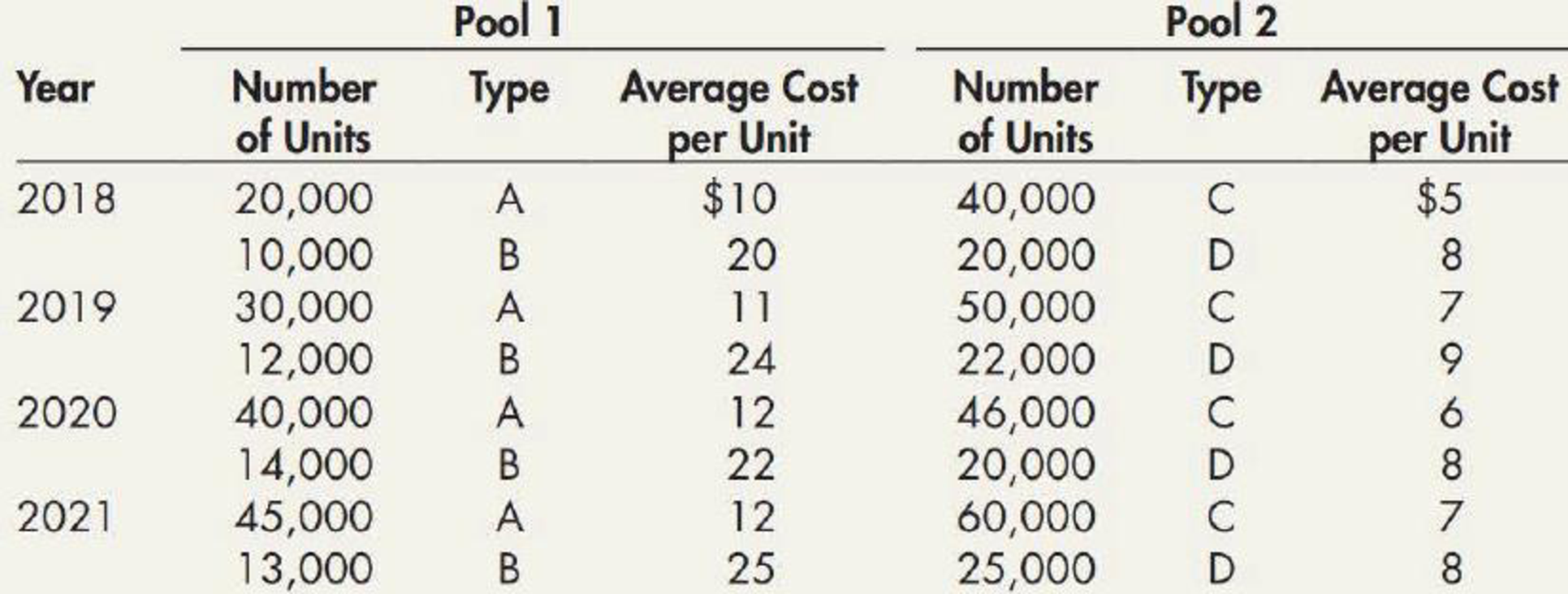Chapter 7, Problem 14P### Intermediate Accounting: Reporting...

3rd Edition
James M. Wahlen + 2 others
ISBN: 9781337788281

#### Solutions

Chapter
Section### Intermediate Accounting: Reporting...

3rd Edition
James M. Wahlen + 2 others
ISBN: 9781337788281
Textbook Problem
1 views

# Dollar-Value LIFO—Comprehensive Kelly Company adopted dollar-value LIFO on January 1, 2018, using two inventory pools each of which includes two types of inventory items. Kelly uses the double-extension method to compute the appropriate cost index. The following information about the inventory at the end of each year is available:Required: 1. Compute the cost index for each year for each pool using a base of 100 for each index. Round each cost index to 4 decimal places. 2. Compute the dollar-value LIFO inventory at the end of each year. Round inventory to the nearest dollar.

1.

To determine

Determine the cost index for each year for each pool using a base of 100 for each index.

Explanation

Cost index: Cost index refer to the index which relates the inventory cost of current year with the base year. The cost index is usually prepared with a sample from the total inventory. It is calculate using the formula given below:

Cost Index = Ending inventory at Current year costEnding inventory at Base year cost×100

Determine the cost index for each year for each pool:

 For 2019 Pool 1 Pool 2 =(30,000×$11)+(12,000×$24)(30,000×$10)+(12,000×$20)×100=$618,000$540,000×100=114.4444 =(50,000×$7)+(22,000×$9)(50,000×$5)+(22,000×$8)×100=$548,000$426,000×100=128.6385 For 2020 Pool 1 Pool 2 =(40,000×$12)+(14,000×$22)(40,000×$10)+(14,000×$20)×100=$788,000$680,000×100=115

2.

To determine

Using the dollar value LIFO method compute the ending inventory for all the years.

### Still sussing out bartleby?

Check out a sample textbook solution.

See a sample solution

#### The Solution to Your Study Problems

Bartleby provides explanations to thousands of textbook problems written by our experts, many with advanced degrees!

Get Started